# 1. Summary

I would like to introduce the method of extracting the red line drawn in the following image by image processing using Python and OpenCV.
Furthermore, I would like to introduce how to output the extracted red line coordinate values ​​to a CSV file and how to perform folder processing that processes multiple images in succession.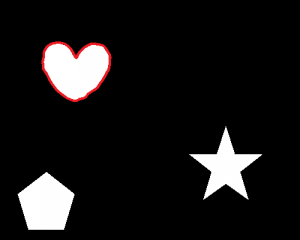# 2. Environment

• OS : Windows10 64bit
• OpenCV :4.1.0
• IDE : Spyder

# 3. Image Processing

Let’s see the python code!

```#import libraries
import numpy as np
from matplotlib import pyplot as plt
import os
import skimage
from skimage import io
from tqdm import tqdm
import glob
import cv2
import csv

#Specify the image file directory to be input and the output destination directory
#process the images in "root folder" - "data folder" - "image folder"
#output into "root folder" - "data folder" - "result folder"
#"root folder" stands for the folder you save the ".py" file in.
path="data/"
input_dir="image"
output_dir="result"

#make full path
all_files=glob.glob(os.path.join(path,input_dir,"**/*.*"),recursive=True)

#create csv file
csvfile = open("np_csv_test.csv", "w")

#loop processing
for i,filepath in tqdm(enumerate(all_files)):

#Define the red color in RGB
#lower = (0, 0, 200)
#upper = (50, 50, 255)
#Note, channel in OpenCV is BGR. And lower, upper threshold needs to be define from Blue, Green, Red respectively.
#You can change these value if you want to.
dst = cv2.inRange(src, (0, 0, 200), (50, 50, 255))

#extract imagefilename.bmp from finename
filename = filepath.rsplit("\\")
#Make full path
outputimage = path + output_dir +"/" + "Extracted_redline_" + filename
cv2.imwrite(outputimage, dst)

#Extract (x,y) coordinate (value) from RED lines.
#Note, in this script, np.where() is used.
#Merge X and Y with np.dstack() method because the return from np.where() is separated.
#indices = np.dstack(np.where(dst == 255)) #This is going to be an error, I don't know why.
indices = np.where(dst == 255) #Return values are Y,X in sequence. Be careful if you want to make an graph in EXCEL.

outputcsv = path + output_dir +"/" + "result.csv";

#Note, eveytime you execute, csv file will be overwritten.
y = indices
y1 = y.reshape(1, y.size)
x = indices
x1 = x.reshape(1, x.size)
axis = np.arange(x.size)
axis = axis.reshape(1, axis.size)
with open(outputcsv, 'ab') as f:
np.savetxt(f, filepath.rsplit("\\"), fmt="%s", delimiter = ",")
np.savetxt(f, axis, fmt="%.0d", delimiter = ",")
np.savetxt(f, x1, fmt="%.0d", delimiter = ",")
np.savetxt(f, y1, fmt="%.0d", delimiter = ",")

#Close csv file
csvfile.close()
```

# 4. Result

## 4.1 Input images

A heart, star, pentagon-shaped with red line will be processed.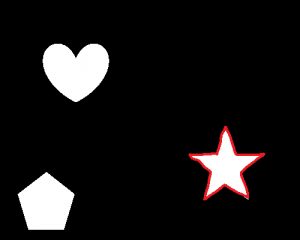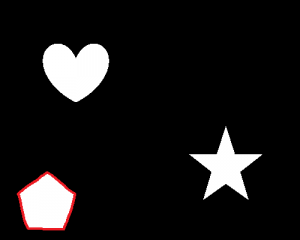## 4.2 Extracted result

The red line on the heart, star, pentagon-shaped is extracted.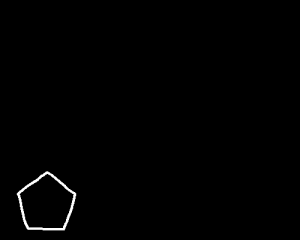## 4.3 Exported result

The coordinates (X, Y) of each pixel that forms the line are output, so it is the same as the image.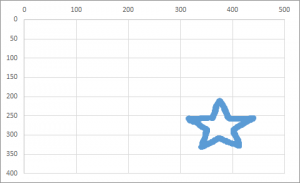シェアする